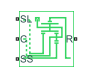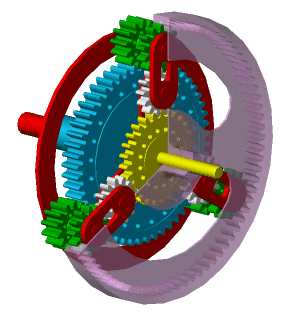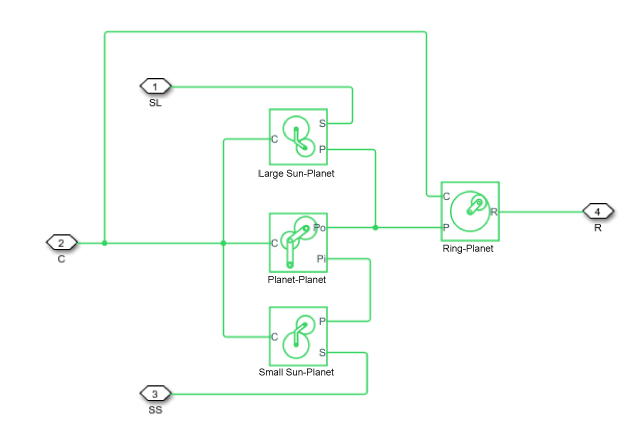# Ravigneaux Gear

Planetary gear with two sun gears and two planet gear sets

• Library:
• Simscape / Driveline / Gears

•## Description

The Ravigneaux Gear block represents a planetary gear train with dual sun and planet gear sets. The two sun gears are centrally located and separated longitudinally along a common rotation axis. The smaller of these gears engages an inner planet gear set, which in turn engages an outer planet gear set. The outer planet gear set, whose length spans the distance between the two sun gears, engages both the larger sun gear and the ring gear.

A carrier holds the planet gear sets in place at different radii. The carrier, which rigidly connects to a drive shaft, can spin as a unit with respect to the sun and ring gears. Revolute joints, each located between a planet gear and the carrier, enable the gears to spin about their individual longitudinal axes.

The relative angular velocities of the sun, planet, and ring gears follow from the kinematic constraints between them. For more information, see Equations.The block models the Ravigneaux gear as a structural component based on the Sun-Planet, Planet-Planet, and Ring-Planet Simscape™ Driveline™ blocks. The figure shows the equivalent block diagram of this structural component.To increase the fidelity of the gear model, you can specify properties such as gear inertia, meshing losses, and viscous losses. By default, gear inertia and viscous losses are assumed to be negligible. The block enables you to specify the inertias of the internal planet gears. To model the inertias of the carrier, large sun, small sun, and ring gears, connect Simscape Inertia blocks to ports C, SL, SS, and R.

### Thermal Model

You can model the effects of heat flow and temperature change by enabling the optional thermal port. To enable the port, set Friction model to ```Temperature-dependent efficiency```.

### Equations

Ideal Gear Constraints and Gear Ratios

The Ravigneaux Gear block imposes four kinematic and four geometric constraints on the four connected axes and the two internal wheels (the inner and outer planet gears):

`${r}_{Ci}{\omega }_{C}={r}_{SS}{\omega }_{SS}+{r}_{Pi}{\omega }_{Pi}$`
`${r}_{Ci}={r}_{SS}+{r}_{Pi}$`
`${r}_{Co}{\omega }_{C}={r}_{SL}{\omega }_{SL}+{r}_{Po}{\omega }_{Po}$`
`${r}_{Co}={r}_{SL}+{r}_{Po}$`
`$\left({r}_{Co}-{r}_{Ci}\right){\omega }_{C}={r}_{Pi}{\omega }_{Pi}+{r}_{Po}{\omega }_{Po}$`
`${r}_{Co}-{r}_{Ci}={r}_{Po}+{r}_{Pi}$`
`${r}_{R}{\omega }_{R}={r}_{Co}{\omega }_{C}+{r}_{Po}{\omega }_{Po}$`
`${r}_{R}={r}_{Co}+{r}_{Po}$`

where:

• rCi is the radius of the inner carrier gear.

• ωC is the angular velocity of the carrier gears.

• ωSS is the angular velocity of the small sun gear.

• rPi is the radius of the inner planet gear.

• ωPi is the angular velocity of the inner planet gear.

• rCo is the radius of the outer carrier gear.

• rSL is the radius of the large sun gear.

• ωSL is the angular velocity of the large sun gear.

• rPo is the radius of the outer planet gear.

• ωPo is the angular velocity of the outer planet gear.

• ωR is the angular velocity of the ring gear.

The ring-to-sun ratios are:

`${g}_{RSS}={r}_{R}/{r}_{SS}={N}_{R}/{N}_{SS}$`

`${g}_{RSL}={r}_{R}/{r}_{SL}={N}_{R}/{N}_{SL}$`

where:

• gRSS is the ring-to-small sun gear ratio.

• NR is the number of teeth in the ring gear.

• NSS is the number of teeth in the small sun gear.

• gRSS is the ring-to-large sun gear ratio.

• NSL is the number of teeth in the large sun gear.

In terms of these gear ratios, the key kinematic constraints are:

`$\left({g}_{RSS}-1\right){\omega }_{C}={g}_{RSS}{\omega }_{R}-{\omega }_{SS}$`

`$\left({g}_{RSL}-1\right){\omega }_{C}={g}_{RSL}{\omega }_{R}-{\omega }_{SL}$`

The six degrees of freedom reduce to two independent degrees of freedom. The gear pairs are (1,2) = (LS,P), (SS,P), (P,R), and (P,P).

Warning

The gear ratio gRSS must be strictly greater than the gear ratio gRSL. The gear ratio gRSL must be strictly greater than one.

The torque transfers are:

`${g}_{RSS}{\tau }_{SS}+{\tau }_{R}-{\tau }_{loss}\left(SS,R\right)=0$`

`${g}_{RSL}{\tau }_{SL}+{\tau }_{R}-{\tau }_{loss}\left(SL,R\right)=0$`

where:

• τSS is the torque transfer for the small sun gear.

• τR is the torque transfer for the ring gear.

• τloss(SS,R) is the torque transfer loss between the small sun gear and the ring gear.

• τSL is the torque transfer for the large sun gear.

• τloss(SL,R) is the torque transfer loss between the large sun gear and the ring gear.

In the ideal case where there is no torque loss, τloss = 0.

Nonideal Gear Constraints and Losses

In the nonideal case, τloss ≠ 0. For more information, see Model Gears with Losses.

### Assumptions and Limitations

• The gears are assumed to be rigid.

## Ports

### Conserving

expand all

Rotational mechanical conserving port associated with the planet gear carrier.

Rotational mechanical conserving port associated with the ring gear.

Rotational mechanical conserving port associated with the large sun gear.

Rotational mechanical conserving port associated with the small sun gear.

Thermal conserving port associated with heat flow. Heat flow affects the power transmission efficiency by altering the gear temperatures.

#### Dependencies

To enable this port, set Friction model to `Temperature-dependent efficiency`.

## Parameters

expand all

### Main

Ratio gRLS of the ring gear to sun gear rotations as defined by the number of ring gear teeth divided by the number of large sun gear teeth.

Ratio, gRSS, of the ring gear wheel to small sun gear rotations as defined by the number of ring gear teeth divided by the number of small sun gear teeth. This gear ratio must be strictly greater than Ring (R) to large sun (SL) teeth ratio (NR/NSL).

### Meshing Losses

Friction model for the block:

• `No meshing losses - Suitable for HIL simulation` — Gear meshing is ideal.

• `Constant efficiency` — Transfer of torque between the gear wheel pairs is reduced by a constant efficiency, η, such that 0 < η ≤ 1.

• `Temperature-dependent efficiency` — Transfer of torque between the gear wheel pairs is defined by the table lookup based on the temperature.

Vector of torque transfer efficiencies, [ηLS, ηSS, ηRP, ηPP], for large sun-planet, small sun-planet, ring-planet, and planet-planet gear wheel pair meshings, respectively. The vector elements must be in the range (0,1].

#### Dependencies

To enable this parameter, set Friction model to `Constant efficiency`.

Vector of temperatures used to construct a 1-D temperature-efficiency lookup table. The vector elements must increase from left to right.

#### Dependencies

To enable this parameter, set Friction model to `Temperature-dependent efficiency`.

Vector of output-to-input power ratios that describe the power flow from the large sun gear to the planet gears, ηLSP. The block uses the values to construct a 1-D temperature-efficiency lookup table.

Each element is an efficiency that relates to a temperature in the Temperature vector. The length of the vector must be equal to the length of the Temperature vector. Each element in the vector must be in the range (0,1].

#### Dependencies

To enable this parameter, set Friction model to ```Temperature-dependent efficiency```.

Vector of output-to-input power ratios that describe the power flow from the small sun gears to the planet gears, ηSSP. The block uses the values to construct a 1-D temperature-efficiency lookup table.

Each element is an efficiency that relates to a temperature in the Temperature vector. The length of the vector must be equal to the length of the Temperature vector. Each element in the vector must be in the range (0,1].

#### Dependencies

To enable this parameter, set Friction model to ```Temperature-dependent efficiency```.

Vector of output-to-input power ratios that describe the power flow from the ring gear to the planet gears, ηRP. The block uses the values to construct a 1-D temperature-efficiency lookup table.

Each element is an efficiency that relates to a temperature in the Temperature vector. The length of the vector must be equal to the length of the Temperature vector. Each element in the vector must be in the range (0,1].

#### Dependencies

To enable this parameter, set Friction model to ```Temperature-dependent efficiency```.

Vector of output-to-input power ratios that describe the power flow from the small sun planet gears to the large sun planet gears, ηPP. The block uses the values to construct a 1-D temperature-efficiency lookup table.

Each element is an efficiency that relates to a temperature in the Temperature vector. The length of the vector must be equal to the length of the Temperature vector. Each element in the vector must be in the range (0,1].

#### Dependencies

To enable this parameter, set Friction model to ```Temperature-dependent efficiency```.

Vector of power thresholds above which the full efficiency factors apply. Enter the thresholds in the order large sun gear, small sun gear, large sun planet gears, and small sun planet gears, all relative to the gear carrier. Below these values, a hyperbolic tangent function smooths the efficiency factor.

When you set Friction model to `Constant efficiency`, the block lowers the efficiency losses to zero when no power is transmitted. When you set Friction model to `Temperature-dependent efficiency`, the block smooths the efficiency factors between zero when at rest and the values provided by the temperature-efficiency lookup tables at the power thresholds.

#### Dependencies

To enable this parameter, set Friction model to ```Temperature-dependent efficiency```.

### Viscous Losses

Vector of viscous friction coefficients, [μLS, μSS, μLSP, μSSP], for the large sun-carrier, small sun-carrier, large sun planet-carrier, and small sun planet-carrier gear motions, respectively.

### Inertia

Inertia model for the block:

• `Off` — Model gear inertia.

• `On` — Neglect gear inertia.

Moment of inertia of the inner planet gear. This value must be positive.

#### Dependencies

To enable this parameter, set Inertia to `On`.

Moment of inertia of the outer planet gear. This value must be positive.

#### Dependencies

To enable this parameter, set Inertia to `On`.

### Thermal Port

To enable these settings, set Friction model to `Temperature-dependent efficiency`.

Thermal energy required to change the component temperature by a single temperature unit. The greater the thermal mass, the more resistant the component is to temperature change.

#### Dependencies

To enable this parameter, set Friction model to ```Temperature-dependent efficiency```.

Block temperature at the start of simulation. The initial temperature sets the initial component efficiencies according to their respective efficiency vectors.

#### Dependencies

To enable this parameter, set Friction model to `Temperature-dependent efficiency`.

expand all

## Version History

Introduced in R2011a